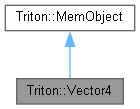Triton Visual simulation library for ocean rendering.
Triton::Vector4 Class Reference

A simple double-precision 4D vector class with no operations defined. More...

`#include <Vector4.h>`

Inheritance diagram for Triton::Vector4:[legend]
Collaboration diagram for Triton::Vector4:[legend]

## Public Member Functions

Vector4 (double px, double py, double pz, double pw)
Constructs a Vector4 from the given x, y, z, and w values. More...

Vector4 (const Vector3 &v3)
Constructs a Vector4 from a Vector3, setting w to 1. More...

Vector4 ()
Default constructor; initializes the Vector4 to (0, 0, 0, 1)

double TRITONAPI Dot (const Vector4 &v) const
Determines the dot product between this vector and another, and returns the result. More...

Vector4 TRITONAPI operator * (double n) const
Scales each x,y,z value of the vector by a constant n, and returns the result. More...

Vector4 TRITONAPI operator * (const Vector4 &v) const
Multiplies the components of two vectors together, and returns the result. More...

Vector4 TRITONAPI operator+ (double n) const
Adds a constant n to each component of the vector, and returns the result. More...

Vector4 TRITONAPI operator - (const Vector4 &v) const
Subtracts the specified vector from this vector, and returns the result. More...

Vector4 TRITONAPI operator+ (const Vector4 &v) const
Adds this vector to the specified vector, and returns the result. More...

## Public Attributes

double x
The x, y, z, and w data members are public for convenience. More...

## Detailed Description

A simple double-precision 4D vector class with no operations defined.

Essentially a struct with constructors.

## ◆ Vector4() [1/2]

 Triton::Vector4::Vector4 ( double px, double py, double pz, double pw )
inline

Constructs a Vector4 from the given x, y, z, and w values.

## ◆ Vector4() [2/2]

 Triton::Vector4::Vector4 ( const Vector3 & v3 )
inline

Constructs a Vector4 from a Vector3, setting w to 1.

## ◆ Dot()

 double TRITONAPI Triton::Vector4::Dot ( const Vector4 & v ) const
inline

Determines the dot product between this vector and another, and returns the result.

## ◆ operator *() [1/2]

 Vector4 TRITONAPI Triton::Vector4::operator * ( double n ) const
inline

Scales each x,y,z value of the vector by a constant n, and returns the result.

## ◆ operator *() [2/2]

 Vector4 TRITONAPI Triton::Vector4::operator * ( const Vector4 & v ) const
inline

Multiplies the components of two vectors together, and returns the result.

## ◆ operator -()

 Vector4 TRITONAPI Triton::Vector4::operator - ( const Vector4 & v ) const
inline

Subtracts the specified vector from this vector, and returns the result.

## ◆ operator+() [1/2]

 Vector4 TRITONAPI Triton::Vector4::operator+ ( double n ) const
inline

Adds a constant n to each component of the vector, and returns the result.

## ◆ operator+() [2/2]

 Vector4 TRITONAPI Triton::Vector4::operator+ ( const Vector4 & v ) const
inline

Adds this vector to the specified vector, and returns the result.

## ◆ x

 double Triton::Vector4::x

The x, y, z, and w data members are public for convenience.

The documentation for this class was generated from the following file: